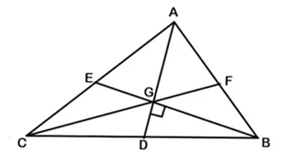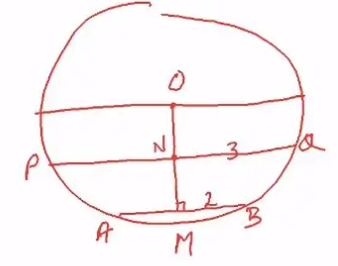### Geometry

Geometry is an important topic that is always covered in MBA exams. Geometry is important because MBA aspirants need to have the diagnostic skill to understand shapes and figures. Some of the topics that are being covered under geometry are circles, triangles, parallel lines. Congruency and Similarity of triangles is a chapter that is being intensively concentrated on geometry.

Below, the details about Geometry under QA for different competitive MBA exams are given: –

CAT :

 Geometry Year No of Questions Good attempt Difficulty 2019 Slot 1 6 4 Moderate Slot 2 6 3 to 4 Moderate 2018 Slot 1 6 to 7 4 to 5 Easy Slot 2 4 2 to 3 Moderate 2017 Slot 1 7 to 8 4 to 5 Moderate Slot 2 7 5 to 6 Easy

XAT :

 Geometry Year No of Questions Good attempt Difficulty 2020 6 4 to 5 Moderate 2019 4 2 to 3 Moderate-Difficult 2018 6 3 Moderate-Difficult

SNAP :

 Geometry Year No of Questions Good attempt Difficulty 2019 6 4 to 5 Easy 2018 7 3 to 4 Moderate 2017 7 3 to 4 Moderate to Difficult

### List of Concepts in that Chapter

The list of concepts that are covered in the Geometry chapter is as follows: –

• Basic Properties of Triangles: – Some basic properties are given below: –
1. The sum of the three interior angles is 180 degree
2. The measure of an exterior angle is equal to the sum of the measures of two remote interior angles
3. The sum of the three exterior angles is 360 degree
4. The side opposite to the greatest angle is the longest, and the side opposite to the smallest angle is the shortest
• Types/Sides of Triangles: For e.g: – “Consider an obtuse triangle with sides 8cm, 15cm & x cm. How many such triangles are possible?”
• Congruency/Similarity of triangles: -For e.g: – “How many noncongruent triangles are possible such that their sides are integers, and the perimeter is equal to 14.”
• Some Important theorems/formula/methods to study: –
1. Angle Bisector Theorem
2. Pythagoras Theorem
3. Midpoint Theorem
4. Basic Proportionality Theorem
5. Heron’s Formula
6. Trigonometric Formulas
7. Determinant Method
• Some Important Lines and Points to Study: –

Altitude

Orthocentre

Perpendicular Bisector

Circumcentre

Median

1. Centroid
2. Angle Bisector
3. Incentre
• Find the angle/sum of angles of the shape inscribed in another shape
• Find the length of a side/sides
• Find the area of the shape, or shape inscribed within another shape

### Some Questions from Previous Papers

Some of the important CAT questions on Geometry that appeared in the previous papers are: –

1. Let A and B be two regular polygons having a and b sides, respectively. If b = 2a and each interior angle of B is 3/2 times each interior angle of A, then each interior angle, in degrees, of a regular polygon with a + b sides is? (CAT 2019 – SLOT 2)

Each interior angle of n sided polygon = (n-2)*180/n
(b-2)*180/b = 3/2 (a-2)*180/a and b = 2a
So, 2a-2/2 = (a-2)*3/2
2a – 2 = 3a – 6
a = 4 and b = 8
So, a + b = 12, a 12-sided polygon
Each interior angle = (12-2)*180/12 = 150 degrees

2. Let ABC be a right-angled triangle with hypotenuse BC of length 20 cm. If AP is perpendicular to BC, then the maximum possible length of AP, in cm, is? (CAT 2019 – SLOT 2)

Let AB = a and AC = b
A^2 + b^2 = 400 by pythagoras
Let AP = x and BP = y, so CP= 20-y
So, by Pythagoras
X^2 + y^2 = a^2 and b^2 = x^2 + (20-y)^2
Maximum possible value of AP occurs when a = b, so a= b=10 root 2
On solving, we get AP = 10 units

3. In a triangle, ABC, medians AD and BE are perpendicular to each other and have lengths 12 cm and 9 cm, respectively. Then, the area of triangle ABC, in sq. cm is? (CAT 2019 – SLOT 2)Draw the third median CF. We know that the :
1. The intersection point of medians i.e. centroid (G) divides each median into 2:1.
2. All three medians divide the triangle into 6 triangles having equal area.
So Area of Triangle ABC = 6* Area of one small triangle—————-1)
Now Given AD = 12 and GD:AG = 1:2 So GD = 1/3 *12 =4
BE = 9 and BG:GE = 2:1 So BG = 2/3 *9 = 6
As BG is perpendicular to GD,
Area of triangle BGD = ½ ×GB × GD = ½ × 6 × 4 =12
Hence, from eq 1) area of triangle ABC = 6 × 12 = 72

4. Let ABCD be a rectangle inscribed in a circle of a radius 13 cm. Which one of the following pairs can represent, in cm, the possible length and breadth of ABCD? (CAT 2018 – SLOT 1)

As ABCD is a rectangle angles A,B,C and D will be 90°. Thus, AC will be diameter of the circle of length 13*2 = 26
So length, width, and 26 will form a Pythagorean triplet. From given options,
only option d) 24, 10 satisfy this condition as

10^2+24^2=26^2

5. In a circle, two parallel chords on the same side of diameter have lengths 4 cm and 6 cm. If the distance between these chords is 1 cm, then the radius of the circle, in cm, is? (CAT 2018 – SLOT 1)### How to deal with the topic preparation

Level 1

• Students are supposed to have basic knowledge of the properties of different shapes/lines-triangles, circle, rectangles, polygons, parallel lines, perpendicular lines, etc.
• Students should develop diagnostic skills to draw and find solutions
• Students need to have the skill to identify which concept/method/formula/theorem to use

Level 2

• Topics such as congruency/similarity of triangles, shape inscribed inside another shape, theorems are focused intensively. So, students need to prepare well and practice problems relating to these topics
• The geometry section in the QA is one of the easiest sections to attempt if the students have done their part properly

Level 3

Students need to look into problems that use multiple concepts to find a solution. Try to answer questions from the advanced level of previous CAT papers, mock tests, and materials. And try to attempt these questions in a time-based manner. Taking too much time for advanced questions will be disadvantageous in cracking the exam. Finally, students need to practice as many questions as they can to get a hold of the concepts and find solutions fast. Practice will always help the student to crack the exam.